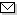Language:   Search:   Contact# Search and Browse the MSC 2000

Search:
Please note that in the second half of 2009, MSC2010 is deployed in the production of ZMATH and will become official standard in January 2010.

Enter a query and click »Search«...

41-XX Approximations and expansions {For all approximation theory in the complex domain, see 30Exx, 30E05 and 30E10; for all trigonometric approximation and interpolation, see 42Axx, 42A10 and 42A15; for numerical approximation, see 65Dxx} → ZMATH
41-00 General reference works (handbooks, dictionaries, bibliographies, etc.) → ZMATH
41-01 Instructional exposition (textbooks, tutorial papers, etc.) → ZMATH
41-02 Research exposition (monographs, survey articles) → ZMATH
41-03 Historical (must also be assigned at least one classification number from Section 01) → ZMATH
41-04 Explicit machine computation and programs (not the theory of computation or programming) → ZMATH
41-06 Proceedings, conferences, collections, etc. → ZMATH
41A10 Approximation by polynomials {For approximation by trigonometric polynomials, see 42A10} → ZMATH
41A15 Spline approximation → ZMATH
41A17 Inequalities in approximation (Bernstein, Jackson, Nikol\cprime ski\u\i-type inequalities) → ZMATH
41A20 Approximation by rational functions → ZMATH
41A25 Rate of convergence, degree of approximation → ZMATH
41A27 Inverse theorems → ZMATH
41A28 Simultaneous approximation → ZMATH
41A29 Approximation with constraints → ZMATH
41A30 Approximation by other special function classes → ZMATH
41A35 Approximation by operators (in particular, by integral operators) → ZMATH
41A36 Approximation by positive operators → ZMATH
41A40 Saturation → ZMATH
41A44 Best constants → ZMATH
41A45 Approximation by arbitrary linear expressions → ZMATH
41A46 Approximation by arbitrary nonlinear expressions; widths and entropy → ZMATH
41A50 Best approximation, Chebyshev systems → ZMATH
41A52 Uniqueness of best approximation → ZMATHAbel prize 2010I. M. Gelfand 1913-2009MSC2010